# Absolute Deviation and Absolute Mean Deviation using NumPy | Python

Absolute value:
Absolute value or the modulus of a real number x is the non-negative value of x without regard to its sign. For example absolute value of 7 is 7 and the absolute value of -7 is also 7.

Deviation:
Deviation is a measure of the difference between the observed value of a variable and some other value, often that variable’s mean.

Absolute Deviation:
The absolute deviation of an element of a data set is the absolute difference between that element and a given point. The absolute deviation of the observations X1, X2, X3, ….., Xn around a value A is defined as –

For discrete (ungrouped) data-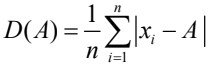For continuous (ungrouped) data-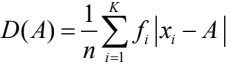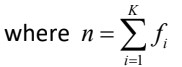Absolute mean deviation:
The absolute mean deviation measures the spread and scatteredness of data around, preferably the median value, in terms of absolute deviation. The absolute deviation of observation X1, X2, X3, ……, Xn is minimum when measured around median i.e. A is the median of the data. Then, thus obtained absolute deviation is termed as the absolute mean deviation and is defined as:

For discrete (ungrouped) data –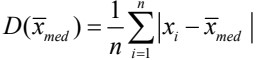For continuous (ungrouped) data –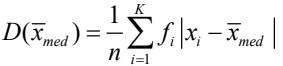Decision Making:

1. The data set having a higher value of absolute mean deviation (or absolute deviation) has more variability.
2. The data set with a lower value of absolute mean deviation (or absolute deviation) is preferable.
–> If there are two data sets with absolute mean values AMD1 and AMD2, and AMD1>AMD2 then the data in AMD1 is said to have more variability than the data in AMD2.

Example:
Following are the number of candidates enrolled each day in last 20 days for the GeeksforGeeks -DS & Algo course –
75, 69, 56, 46, 47, 79, 92, 97, 89, 88, 36, 96, 105, 32, 116, 101, 79, 93, 91, 112

Code #1: Absolute deviation using numpy

 `# Importing mean, absolute from numpy ` `from` `numpy ``import` `mean, absolute ` ` `  `data ``=` `[``75``, ``69``, ``56``, ``46``, ``47``, ``79``, ``92``, ``97``, ``89``, ``88``, ` `        ``36``, ``96``, ``105``, ``32``, ``116``, ``101``, ``79``, ``93``, ``91``, ``112``] ` ` `  `# Assume any point A about which  ` `# absolute deviation is to be calculated ` `A ``=` `79` ` `  `sum` `=` `0`  `# Initialize sum to 0 ` ` `  `# Absolute deviation calculation ` ` `  `for` `i ``in` `range``(``len``(data)): ` `    ``av ``=` `absolute(data[i] ``-` `A)   ``# Absolute value of the differences  ` `                                 ``# of each data point and A ` ` `  `    ``# Summing all those absolute values ` `    ``sum` `=` `sum` `+` `av                ` ` `  `# Sum divided by length of data yields ` `# the absolute deviation ` `print``(``sum` `/` `len``(data))              `

Output:

`20.15`

Code #2: Absolute mean deviation using numpy

 `# Importing mean, absolute from numpy ` `from` `numpy ``import` `mean, absolute ` ` `  `data ``=` `[``75``, ``69``, ``56``, ``46``, ``47``, ``79``, ``92``, ``97``, ``89``, ``88``,  ` `        ``36``, ``96``, ``105``, ``32``, ``116``, ``101``, ``79``, ``93``, ``91``, ``112``] ` ` `  `# Absolute mean deviation ` `mean(absolute(data ``-` `mean(data))) `

Output:

`20.055`

Code #3: Absolute mean deviation using pandas

 `# Import the pandas library as pd ` `import` `pandas as pd ` ` `  `data ``=` `[``75``, ``69``, ``56``, ``46``, ``47``, ``79``, ``92``, ``97``, ``89``, ``88``, ` `        ``36``, ``96``, ``105``, ``32``, ``116``, ``101``, ``79``, ``93``, ``91``, ``112``] ` ` `  `# Creating data frame of the given data ` `df ``=` `pd.DataFrame(data) ` ` `  `# Absolute mean deviation ` `df.mad()    ``# mad() is mean absolute deviation function `

Output:

`20.055`

My Personal Notes arrow_drop_upCheck out this Author's contributed articles.

If you like GeeksforGeeks and would like to contribute, you can also write an article using contribute.geeksforgeeks.org or mail your article to contribute@geeksforgeeks.org. See your article appearing on the GeeksforGeeks main page and help other Geeks.

Please Improve this article if you find anything incorrect by clicking on the "Improve Article" button below.